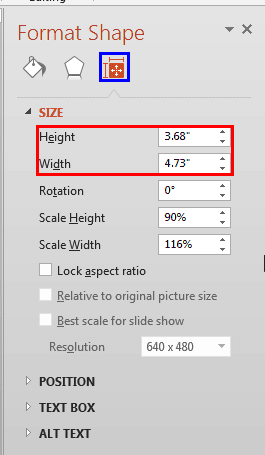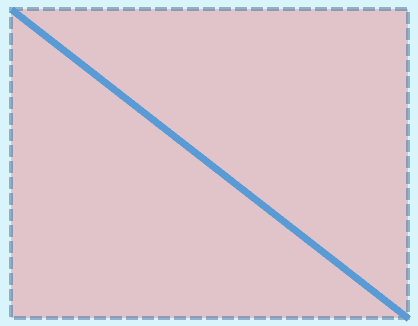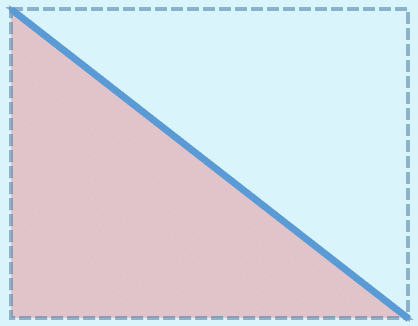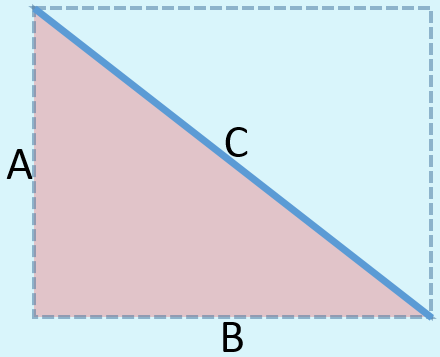# Finding Length of a Diagonal Line in PowerPoint 2013 for Windows

Learn how to find out the length of a slanted (diagonal) line in PowerPoint 2013 for Windows. We get some help from an ancient philosopher.

Author:

Product/Version: PowerPoint 2013 for Windows

OS: Microsoft Windows 7 and higher

Sometimes, when you draw a line in PowerPoint that is slanted or sloped, you may thereafter want to know the length of your line. Believe it or not, there is no option to do this within the program! Look at Figure 1, where you can see a straight line drawn in PowerPoint 2013 for Windows. Yes, the line is straight but is also a diagonal line.Figure 1:A diagonal line on a PowerPoint slide

We now right-click the line to bring up the contextual menu you see in Figure 2. Within this menu, select the Format Shape option.Figure 2: Format Shape option within the right-click contextual menu

This brings up the Format Shape Task Pane as shown in Figure 3. Now, click the Size & Properties button (highlighted in blue within Figure 3) and you see both Height and a Width values (highlighted in red within Figure 3) but no Length value!Figure 3: Format Shape Task Pane includes Height and Width values

You might be curious about how a line can have a Height and a Width rather than just a Length?

Tip: Want to change a diagonal line to a straight horizontal or vertical line? Look at our Change a Diagonal Line to Horizontal / Vertical Line in PowerPoint 2013 for Windows tutorial.

The actual answer is that these values are not for the line but an imaginary rectangle that spans the line. In Figure 4, you can see that we have placed this imaginary rectangle behind the line. The Height and Width you saw within the Format Shape dialog box in Figure 3, earlier on this page pertain to this rectangle!Figure 4: Imaginary rectangle that contains the diagonal line

Look closely at Figure 4, and you will notice that the diagonal line in question not only created an imaginary rectangle, but it also created two imaginary right-angled triangles that are exactly the same. When you draw a diagonal line connecting two opposite corners of a rectangle, you end up creating the imaginary triangles shown in Figure 5, below.Figure 5: Two imaginary right-angled triangles are created with a diagonal line

The Width and Height values that we saw within the Format Shape Task Pane (see Figure 3 shown previously on this page) thus also are the lengths of two sides of any one of the triangles. Since we already know the length of two sides of our triangle, we can easily use a little geometry to find the length of the line we started with!

How many of you remember the Pythagoras' theorem from your school days? If we use the logic behind the Pythagoras' theorem, you will know that A²+B²=C² (A, B, and C are the three sides of our triangle, as shown in Figure 6, below).Figure 6: A, B, and C are the three sides of our imaginary triangle

The values that we know so far are:

A: 3.68
B: 4.73

Thus, 3.68²+4.73²=C²

We actually created an Excel sheet for you that already has these formulas inserted. You just need to type in your values for A and B, and will instantly see the value for C!

Thus the length of our original line is 5.99.

You can similarly use the embedded Excel sheet on this page to find the length of any diagonal line within PowerPoint.

### Popular PostsChange the Unit of Measurement in Windows 10
Learn how to change the unit of measurement from US to Metric, and vice versa in Windows 10.PowerPoint Tutorials, Articles and Reviews
Tutorials, reviews, articles and templates for Microsoft PowerPoint and other presentation software.PowerPoint Templates
Find over 6000 PowerPoint templates in thousands of categories and all colors—from a trusted PowerPoint template resource for 20 years.Create Animated GIFs from PowerPoint Slides
Explore ways to create animated GIFs from PowerPoint slides.

## Polygon Center Circles for PowerPoint

These special circles have polygon centers: the centers are made of triangles, squares, pentagons, and hexagons! And based on the sides of the polygon, the rest of the circle has that many segments.

This is the original page. An AMP (Accelerated Mobile Page) version of this page is also available for those on mobile platforms, at Finding Length of a Diagonal Line in PowerPoint 2013 for Windows.

Microsoft and the Office logo are trademarks or registered trademarks of Microsoft Corporation in the United States and/or other countries.

Plagiarism will be detected by Copyscape